Description: test createpts

The objective is to add points to a mesh using the createpts command. The test consists of eight examples each exercising the command in a specific geometry (rectangular, cylindrical, spherical) with some variations on input parameters. A geometry is defined in one of the coordinate systems. The createpts command is used to create a point distribution within the geometry. The output consists of one gmv file for each example.

Individual Descriptions

Example 3

createpts/rtp

spherical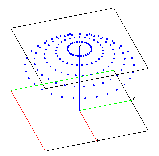Example 4

createpts/brick/xyz

material interface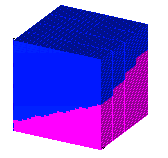Example 2

createpts/rtz

(cylindrical)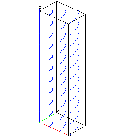Example 1

createpts/xyz

(rectangular)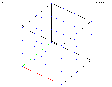Example 5

createpts/sphere/diamond

partial sphere using region boundaries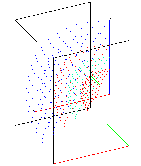Example 6

createpts/sphere

full sphere using region boundaries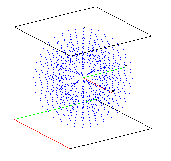Example 7

createpts/brick/xyz

brick mesh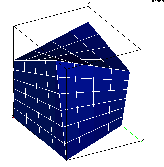Example 8

createpts/sphere

gridding a sphere, including materials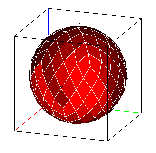Example 9

createpts/random/xyz

random points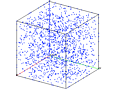Next Demo